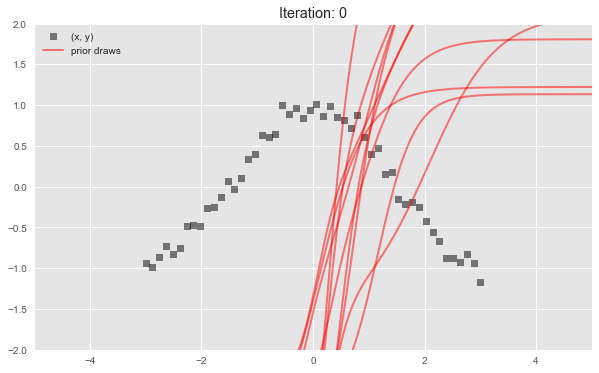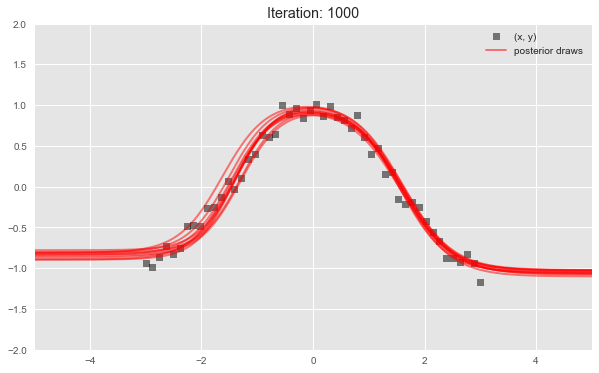Probabilistic modeling in Edward uses a simple language of random variables. Here we will show a Bayesian neural network. It is a neural network with a prior distribution on its weights.

A webpage version is available at http://edwardlib.org/getting-started.

In :
%matplotlib inline
from __future__ import absolute_import
from __future__ import division
from __future__ import print_function

import edward as ed
import matplotlib.pyplot as plt
import numpy as np
import tensorflow as tf

from edward.models import Normal

plt.style.use('ggplot')

In :
def build_toy_dataset(N=50, noise_std=0.1):
x = np.linspace(-3, 3, num=N)
y = np.cos(x) + np.random.normal(0, noise_std, size=N)
x = x.astype(np.float32).reshape((N, 1))
y = y.astype(np.float32)
return x, y

def neural_network(x, W_0, W_1, b_0, b_1):
h = tf.tanh(tf.matmul(x, W_0) + b_0)
h = tf.matmul(h, W_1) + b_1
return tf.reshape(h, [-1])


First, simulate a toy dataset of 50 observations with a cosine relationship.

In :
ed.set_seed(42)

N = 50  # number of data points
D = 1   # number of features

x_train, y_train = build_toy_dataset(N)


Next, define a two-layer Bayesian neural network. Here, we define the neural network manually with tanh nonlinearities.

In :
W_0 = Normal(loc=tf.zeros([D, 2]), scale=tf.ones([D, 2]))
W_1 = Normal(loc=tf.zeros([2, 1]), scale=tf.ones([2, 1]))
b_0 = Normal(loc=tf.zeros(2), scale=tf.ones(2))
b_1 = Normal(loc=tf.zeros(1), scale=tf.ones(1))

x = x_train
y = Normal(loc=neural_network(x, W_0, W_1, b_0, b_1),
scale=0.1 * tf.ones(N))


Next, make inferences about the model from data. We will use variational inference. Specify a normal approximation over the weights and biases.

In :
qW_0 = Normal(loc=tf.get_variable("qW_0/loc", [D, 2]),
scale=tf.nn.softplus(tf.get_variable("qW_0/scale", [D, 2])))
qW_1 = Normal(loc=tf.get_variable("qW_1/loc", [2, 1]),
scale=tf.nn.softplus(tf.get_variable("qW_1/scale", [2, 1])))
qb_0 = Normal(loc=tf.get_variable("qb_0/loc", ),
scale=tf.nn.softplus(tf.get_variable("qb_0/scale", )))
qb_1 = Normal(loc=tf.get_variable("qb_1/loc", ),
scale=tf.nn.softplus(tf.get_variable("qb_1/scale", )))


Defining tf.get_variable allows the variational factors’ parameters to vary. They are initialized randomly. The standard deviation parameters are constrained to be greater than zero according to a softplus) transformation.

In :
# Sample functions from variational model to visualize fits.
rs = np.random.RandomState(0)
inputs = np.linspace(-5, 5, num=400, dtype=np.float32)
x = tf.expand_dims(inputs, 1)
mus = tf.stack(
[neural_network(x, qW_0.sample(), qW_1.sample(),
qb_0.sample(), qb_1.sample())
for _ in range(10)])

In :
# FIRST VISUALIZATION (prior)

sess = ed.get_session()
tf.global_variables_initializer().run()
outputs = mus.eval()

fig = plt.figure(figsize=(10, 6))
ax.set_title("Iteration: 0")
ax.plot(x_train, y_train, 'ks', alpha=0.5, label='(x, y)')
ax.plot(inputs, outputs.T, 'r', lw=2, alpha=0.5, label='prior draws')
ax.plot(inputs, outputs[1:].T, 'r', lw=2, alpha=0.5)
ax.set_xlim([-5, 5])
ax.set_ylim([-2, 2])
ax.legend()
plt.show()Now, run variational inference with the Kullback-Leibler divergence in order to infer the model’s latent variables with the given data. We specify 1000 iterations.

In :
inference = ed.KLqp({W_0: qW_0, b_0: qb_0,
W_1: qW_1, b_1: qb_1}, data={y: y_train})
inference.run(n_iter=1000, n_samples=5)

1000/1000 [100%] ██████████████████████████████ Elapsed: 12s | Loss: -5.755

Finally, criticize the model fit. Bayesian neural networks define a distribution over neural networks, so we can perform a graphical check. Draw neural networks from the inferred model and visualize how well it fits the data.

In :
# SECOND VISUALIZATION (posterior)

outputs = mus.eval()

fig = plt.figure(figsize=(10, 6))
ax.set_title("Iteration: 1000")
ax.plot(x_train, y_train, 'ks', alpha=0.5, label='(x, y)')
ax.plot(inputs, outputs.T, 'r', lw=2, alpha=0.5, label='posterior draws')
ax.plot(inputs, outputs[1:].T, 'r', lw=2, alpha=0.5)
ax.set_xlim([-5, 5])
ax.set_ylim([-2, 2])
ax.legend()
plt.show()The model has captured the cosine relationship between $x$ and $y$ in the observed domain.